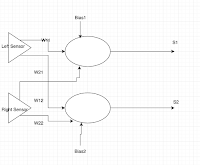Neuron Controlled Edge follower updated

In the last post experimentation with an artificial neuron controlling the Bit:Bot to follow the edge of a line (it follows the left-hand side of the line).

More details can be found in the previous post.The neurons (well two separate ones, S1 and S2) are produced using weighted sums - summing the weights x inputs [ right-hand sensor (rs) and left-hand sensor (ls)] plus a bias for each neuron in this case w and w.
net=w+w*rs+w*ls
net2=w+w*rs+w*ls

If weighted sum >=0 then its output 1 otherwise 0

What actual causes S1 to be either 1 or 0 is all defined by a set of weights w (three for the first neurone, S1,  three for S2).

w=[0,-1,1,-1,1,-1]

Modifications to the code in the last post have been around fine tuning the values in converting the outputs of the two neurons S1 and S2 into actions as shown below.
if s1==1 and s2==1:
forward(20)
elif s1==0 and s2==1:
forward(15)
right_turn(25)
elif s1==1 and s2==0:
forward(15)
left_turn(25)
elif s1==0 and s2==0:
backward(5)

The functions for forward, right_turn, etc are defined elsewhere.

To change the function of the system, change the values in wThe complete code is shown below.

Code
from microbit import *
import neopixel, random, array

w=[]

def forward(n):
pin0.write_analog(551)
pin8.write_digital(0)
pin1.write_analog(551)
pin12.write_digital(0)
sleep(n)

def backward(n):
pin0.write_analog(551)
pin8.write_digital(1)
pin1.write_analog(551)
pin12.write_digital(1)
sleep(n)

def right_turn(n):
pin0.write_analog(511)
pin8.write_digital(0)
pin1.write_analog(511)
pin12.write_digital(1)
sleep(n)

def left_turn(n):
pin0.write_analog(551)
pin8.write_digital(1)
pin1.write_analog(551)
pin12.write_digital(0)
sleep(n)

w=[0,-1,1,-1,1,-1]

while True:

net=w+w*rs+w*ls
net2=w+w*rs+w*ls

if net>=0:
s1=1
else:
s1=0

if net2>=0:
s2=1
else:
s2=0

if s1==1 and s2==1:
forward(20)
elif s1==0 and s2==1:
forward(15)
right_turn(25)
elif s1==1 and s2==0:
forward(15)
left_turn(25)
elif s1==0 and s2==0:
backward(5)

Video of it in action:

Please feel free to use the code and improve on it, and I would especially welcome the seeing the improvement through the comments.

All opinions in this blog are the Author's and should not in any way be seen as reflecting the views of any organisation the Author has any association with. Twitter @scottturneruon

4Tronix Bit:Bot Neuron Controlled Edge follower

In the last post I was playing with 4Tronix's Bit:Bot. In this post I will show the initial experimentation with an artificial neuron controlling the Bit:Bot to follow the edge of a line (it follows the left-hand side of the line).The neurons (well two separate ones, S1 and S2) are produced using weighted sums - summing the weights x inputs [ right-hand sensor (rs) and left-hand sensor (ls)] plus a bias for each neuron in this case w and w.

net=w+w*rs+w*ls
net2=w+w*rs+w*ls

If weighted sum >=0 then its output 1 otherwise 0
if net>=0:
s1=1
else:
s1=0

if net2>=0:
s2=1
else:
s2=0

What actual causes S1 to be either 1 or 0 is all defined by a set of weights w (three for the first neurone, S1,  three for S2).

w=[0,-1,1,-1,1,-1]

Converting the outputs of the two neurones S1 and S2 into actions is shown below.
if s1==1 and s2==1:
forward(40)
elif s1==0 and s2==1:
forward(30)
right_turn(10)
elif s1==1 and s2==0:
forward(30)
left_turn(10)
elif s1==0 and s2==0:
backward(40)

The functions for forward, right_turn, etc are defined elsewhere.

At the moment the movement is a bit rough and it is a little simpler to build a version that follows the centre of the line; this approach though, works with thinner lines.

To change the function of the system, change the values in w; for example to produce one that follows the centre of the line just change w (I will leave that to someone to work on). The complete code is shown below.

Code
from microbit import *
import neopixel, random, array

w=[]

def forward(n):
pin0.write_analog(551)
pin8.write_digital(0)
pin1.write_analog(551)
pin12.write_digital(0)
sleep(n)

def backward(n):
pin0.write_analog(551)
pin8.write_digital(1)
pin1.write_analog(551)
pin12.write_digital(1)
sleep(n)

def right_turn(n):
pin0.write_analog(511)
pin8.write_digital(0)
pin1.write_analog(511)
pin12.write_digital(1)
sleep(n)

def left_turn(n):
pin0.write_analog(551)
pin8.write_digital(1)
pin1.write_analog(551)
pin12.write_digital(0)
sleep(n)

w=[0,-1,1,-1,1,-1]

while True:

net=w+w*rs+w*ls
net2=w+w*rs+w*ls

if net>=0:
s1=1
else:
s1=0

if net2>=0:
s2=1
else:
s2=0

if s1==1 and s2==1:
forward(40)
elif s1==0 and s2==1:
forward(30)
right_turn(10)
elif s1==1 and s2==0:
forward(30)
left_turn(10)
elif s1==0 and s2==0:
backward(40)

All opinions in this blog are the Author's and should not in any way be seen as reflecting the views of any organisation the Author has any association with. Twitter @scottturneruon

4Tronix Bit:Bot - now there is a good idea.

When I first heard of this robot, my first thought was what a great idea; a robot with neopixels (I know I should be saying 'smart RGB LEDs' but neopixels is so much more snappier) controlled via a micro:bit.

A good starting point for learning more about this robot, is the details on the 4Tronix site/blog, which includes build guidance and programming instructions for micropython and PXT. Though for the micropython code you might need to change pinX.digital_write() to pinX.write_digital() where X is the pin number.

My play code was to randomly select which neopixels to light up, I didn't include code to turn them off so multiple ones can be on. The robot is driven forwards, waits, backward, waits, turns to the right and then the left; and then repeats.

Code:
from microbit import *
import neopixel, random

np = neopixel.NeoPixel(pin13, 12)

def forward(n):
pin0.write_digital(1)
pin8.write_digital(0)
pin1.write_digital(1)
pin12.write_digital(0)
sleep(n*1000)

def halt(n):
pin0.write_digital(0)
pin8.write_digital(0)
pin1.write_digital(0)
pin12.write_digital(0)
sleep(n*1000)

def backward(n):
pin0.write_digital(0)
pin8.write_digital(1)
pin1.write_digital(0)
pin12.write_digital(1)
sleep(n*1000)

def right_turn(n):
pin0.write_digital(1)
pin8.write_digital(0)
pin1.write_digital(0)
pin12.write_digital(1)
sleep(n*1000)

def left_turn(n):
pin0.write_digital(0)
pin8.write_digital(1)
pin1.write_digital(1)
pin12.write_digital(0)
sleep(n*1000)

while True:
pxl=random.randint(1,11)
rd=random.randint(1,32)
gr=random.randint(1,32)
bl=random.randint(1,32)
t1=random.randint(10,100)
np[pxl] = (rd, gr, bl)
np.show()
forward(1)
halt(1)
backward(1)
halt(1)
right_turn(1)

left_turn(1)

The video below shows it in action, the code is simple but this is a lovely robot to program especially if the mu editor is used.

All opinions in this blog are the Author's and should not in any way be seen as reflecting the views of any organisation the Author has any association with. Twitter @scottturneruon